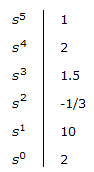# Electronics and Communication Engineering - Automatic Control Systems - Discussion

1.

The first column of a Routh array isHow many roots of the corresponding characteristic equation are in left half s-plane?

 [A]. 2 [B]. 3 [C]. 4 [D]. 5

Explanation:

Total number of roots is 5. Since two sign changes in first column, two roots are in right half plane.

Hence 3 roots in left half plane.

 Pardeep Kumar said: (Nov 8, 2016) How we came to know the number of roots? Please explain.

 Priya said: (Sep 13, 2017) I think it is wrong, because in the routh array there is only one sign change.

 Deswal said: (May 30, 2018) Highest power of s in characteristic equation decides the no of roots. Here it is 5. Also, sign change one time from positive to negative and then again from negative to positive hence it will change twice. So 5-2 =3 is equal to left half poles.

 Aishwarya said: (Sep 28, 2019) There are only two sign changes hence 2 roots in the left half of s-plane the how option B is correct can you explain it in detail?